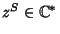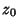## Symmetric Points

Two pointsandare symmetric with respect to a Circle or straight Lineif all Circles and straight Lines passing throughandare orthogonal to. Möbius Transformations preserve symmetry. Let a straight line be given by a pointand a unit Vector, thenLet a Circle be given by centerand Radius, then